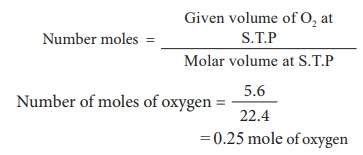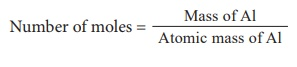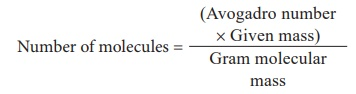Home | | Science 10th Std | Solved Problems

# Solved Problems

I. Calculation of molar mass II. Calculation based on number of moles from mass and volume III. Calculation of mass from mole IV. Calculation based on number of atoms/ molecules. V. Calculation based on molar volume VI. Calculation based on % composition

Atoms and Molecules (Science)

SOLVED PROBLEMS

## I. Calculation of molar mass

Calculate the gram molar mass of the Â­following.

1) H2O        2) CO2        3) Ca3 (PO4)2

Solution:

1) H2O

Atomic masses of H = 1, O = 16

Gram molar mass of H2O

= (1 Ă— 2) + (16 Ă— 1)

= 2 + 16

Gram molar mass of H2O = 18 g

2) CO2

Atomic masses of C = 12, O = 16

Gram molar mass of CO2

= (12 Ă— 1) + (16 Ă— 2)

= 12 + 32

Gram molar mass of CO2 = 44 g 3)

3) Ca3 (PO4)2

Atomic masses of Ca = 40, P = 30, O = 16.

Gram molar mass of Ca3 (PO4)2

= (40 Ă— 3) + [30 + (16 Ă— 4)] Ă— 2

= 120 + (94 Ă— 2)

= 120 + 188

Gram molar mass of Ca3(PO4)2 = 308 g

## II. Calculation based on number of moles from mass and volume

1) Calculate the number of moles in 46 g of sodium?= 46 / 23

2 moles of sodium

2) 5.6 litre of oxygen at S.T.P3) Calculate the number of moles of a sample that contains 12.046 Ă— 1023 atoms of iron ?## III. Calculation of mass from mole

Calculate the mass of the following

1) 0.3 mole of aluminium (Atomic mass of Al = 27)Mass = No. of moles Ă— atomic mass

So, mass of Al = 0.3 Ă— 27

= 8.1 g

2) 2.24 litre of SO2 gas at S.T.P

Molecular mass of SO2 = 32 + (16 Ă— 2)

= 32 + 32 = 64Mass = No. of moles Ă— molecular mass

Mass = 0.1 Ă— 64

Mass of SO2 = 6.4 g

3) 1.51 Ă— 1023 molecules of water

Molecular mass of H2O = 180.25 = mass / 18

Mass = 0.25 Ă— 18

Mass = 4.5 g

4) 5 Ă— 1023 molecules of glucose ?

Molecular mass of glucose = 180= (180 Ă— 5 Ă— 1023) / 6.023 Ă— 1023

= 149.43 g

## IV. Calculation based on number of atoms/ molecules.

1) Calculate the number of molecules in 11.2 litre of CO2 at S.T.P= 11.2 / 22.4

= 0.5 mole

Number of molecules of CO2 = number of moles of CO2 Ă— Avogadroâ€™s number

= 0.5 Ă— 6.023 Ă— 1023

= 3.011 Ă— 1023 molecules of CO2

2) Calculate the number of atoms present in 1 gram of gold (Atomic mass of Au = 198)Number of atoms of Au = 3.042 Ă— 1021 g

3) Calculate the number of molecules in 54 gm of H2O?Number of molecules of water = 6.023 Ă— 1023 Ă— 54 / 18

= 18.069 Ă— 1023 molecules

4) Calculate the number of atoms of oxygen and carbon in 5 moles of CO2.

1 mole of CO2 contains 2 moles of oxygen

5 moles of CO2 contain 10 moles of oxygen

Number of atoms of oxygen = Number of moles of oxygen Ă— Avogadroâ€™s number

= 10 Ă— 6.023 Ă— 1023

= 6.023 Ă— 1024 atoms of Oxygen

1 mole of CO2 contains 1 mole of carbon

5 moles of CO2 contains 5 moles of carbon

No. of atoms of carbon = No.of moles of carbon Ă— Avogadroâ€™s number

= 5 Ă— 6.023 Ă— 1023

= 3.011 Ă— 1024 atoms of Carbon

## V. Calculation based on molar volume

Calculate the volume occupied by:

1) 2.5 mole of CO2 at S.T.P2) 3.011 Ă— 1023 of ammonia gas molecules= 2 moles

Volume occupied by NH3

= number of moles Ă— molar volume

= 2 Ă— 22.4

= 44.8 litres at S.T.P

3) 14 g nitrogen gas

Number of moles = 14 / 28

= 0.5 mole

Volume occupied by N2 at S.T.P

no. of moles Ă— molar volume

0.5 Ă— 22.4

11.2 litres.

## VI. Calculation based on % composition

Calculate % of S in H2SO4

Molar mass of H2SO4

= (1 Ă— 2) + (32 Ă— 1) + (16 Ă— 4)

= 2 + 32 + 64

= 98 gTags : Atoms and Molecules | Science , 10th Science : Chapter 7 : Atoms and Molecules
Study Material, Lecturing Notes, Assignment, Reference, Wiki description explanation, brief detail
10th Science : Chapter 7 : Atoms and Molecules : Solved Problems | Atoms and Molecules | Science